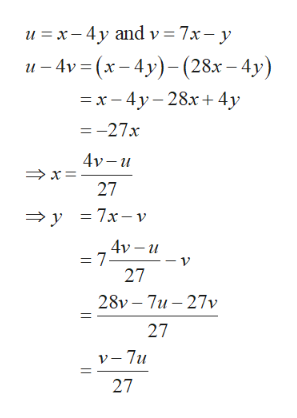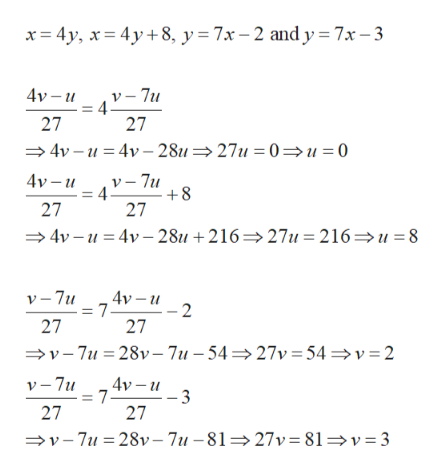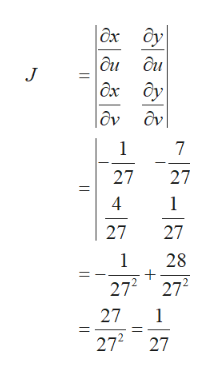# Use the given transformation to evaluate the double integral, 7((x-4y)/(7x-y)) dA where R is the parallelogram enclosed by the lines x − 4y = 0, x − 4y = 8, 7x − y = 2, and 7x − y = 3; u = x − 4y, v = 7x − y

Question
193 views

Use the given transformation to evaluate the double integral, 7((x-4y)/(7x-y)) dA

where R is the parallelogram enclosed by the lines

x − 4y = 0,

x − 4y = 8,

7x − y = 2,
and
7x − y = 3; u = x − 4y, v = 7x − y
check_circle

Step 1

Obtain x and y in terms of u and v as follows.help_outlineImage Transcriptioncloseu x-4y and v= 7x- y u-4v (x-4y)(28&x - 4y) =x-4y-28x+4y =-27x 4v-u 27 y =7x-v 4ν- υ = 7 27 28v 7u 27v 27 v-7u 27 fullscreen
Step 2

The given region R is the parallelogram bounded by the given lines and transform these lines using the obtained x and y as follows.help_outlineImage Transcriptionclosex 4y, x4y8, y = 7x - 2 and y = 7x-3 4v-u 4V-7u 27 27 4v u4v 28u 27u = 0=u =0 4v -u v-7u = 4 +8 27 27 4v u4v 28u +216>27u=216=>u =8 4v -u = 7- 2 27 v-7u 27 v-7u 28v-7u -54>27v 54 v 2 v-7u 4v-u = 7- -3 27 27 v-7u 28v-7u -81= 27v = 81=v=3 fullscreen
Step 3

The region of integration S is bounded by u = 0, u = 8, v = 2 and v = 3. Obtain...help_outlineImage Transcriptioncloseax Oy ди ди дх дv J 1 7 27 4 1 27 1 28 272 27 27 1 272 27 fullscreen

### Want to see the full answer?

See Solution

#### Want to see this answer and more?

Solutions are written by subject experts who are available 24/7. Questions are typically answered within 1 hour.*

See Solution
*Response times may vary by subject and question.
Tagged in

### Math